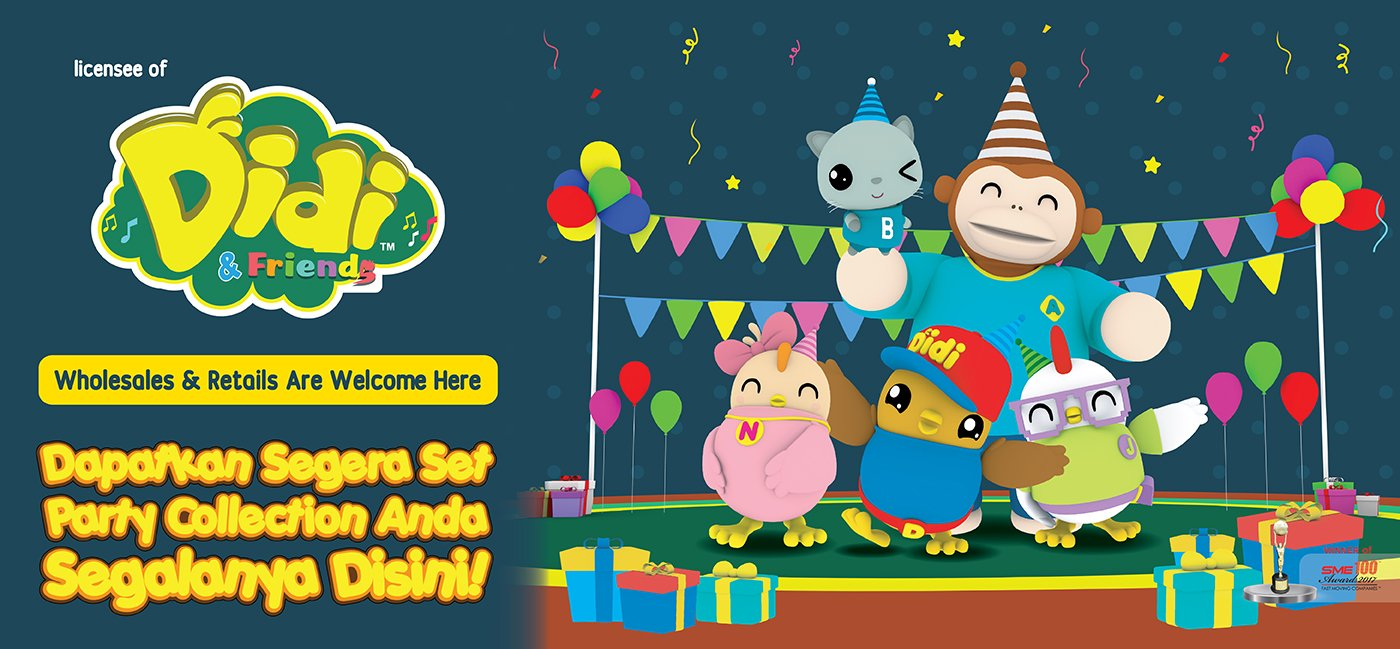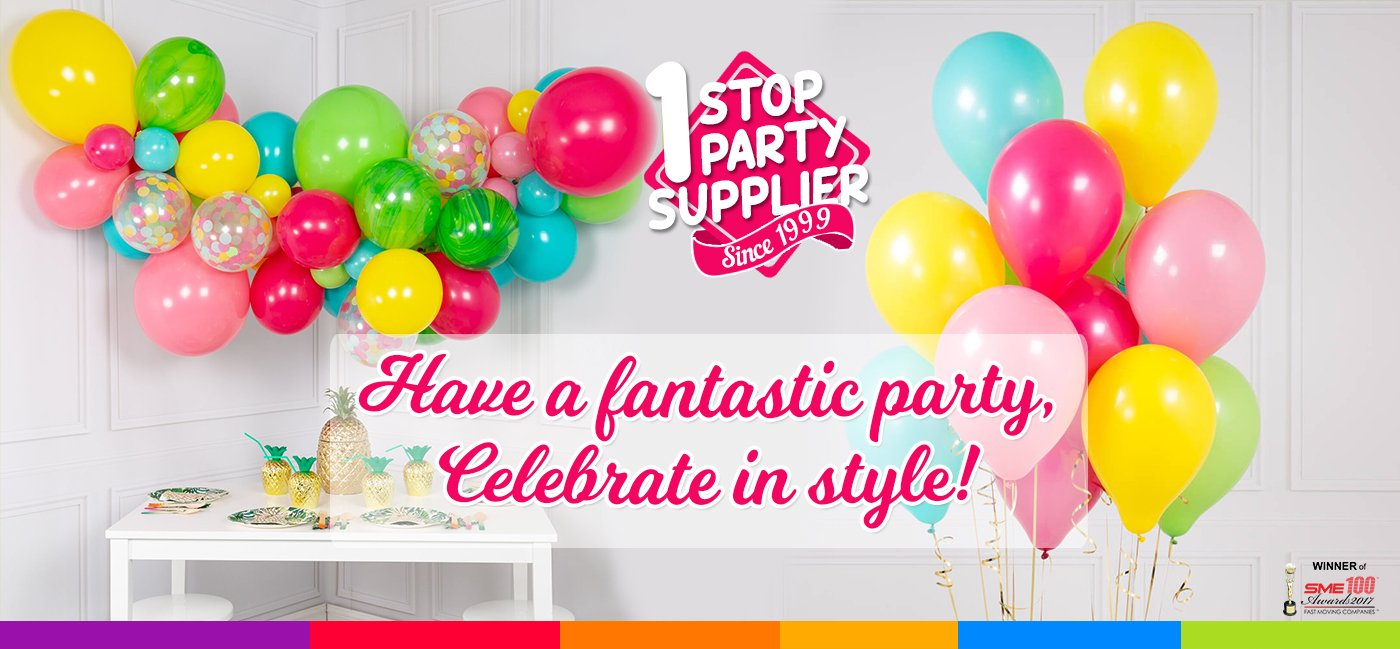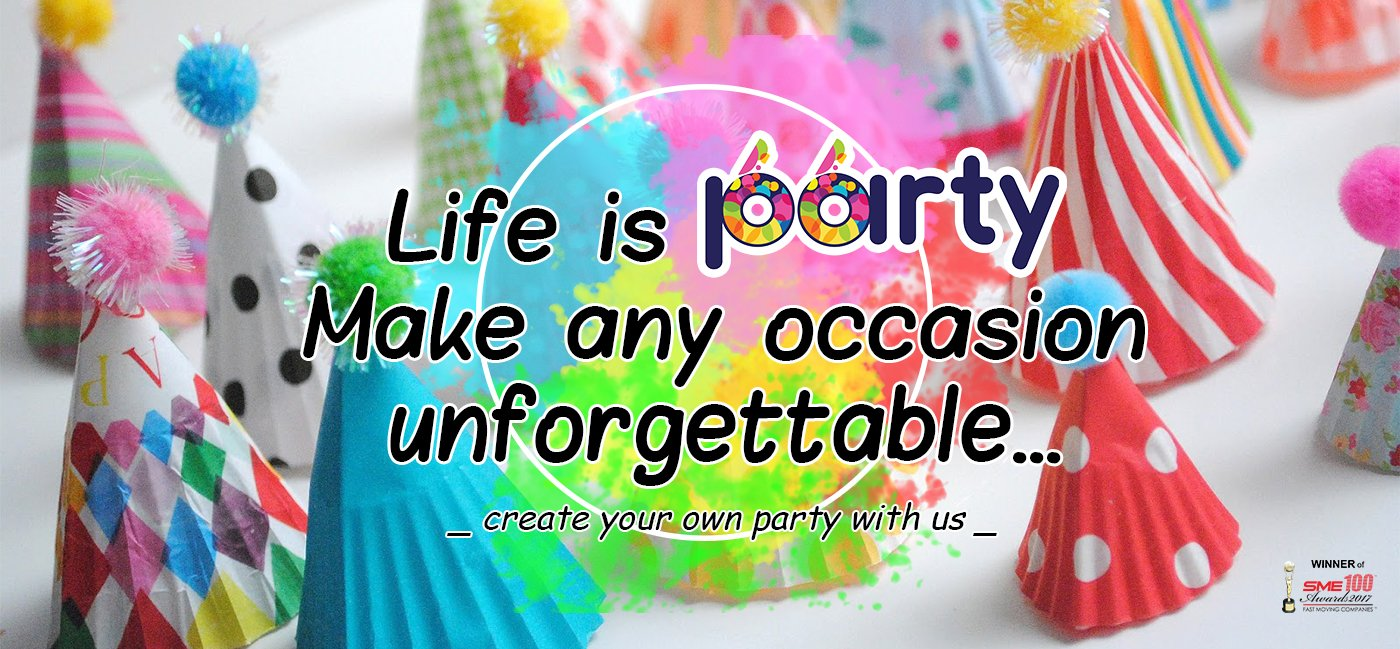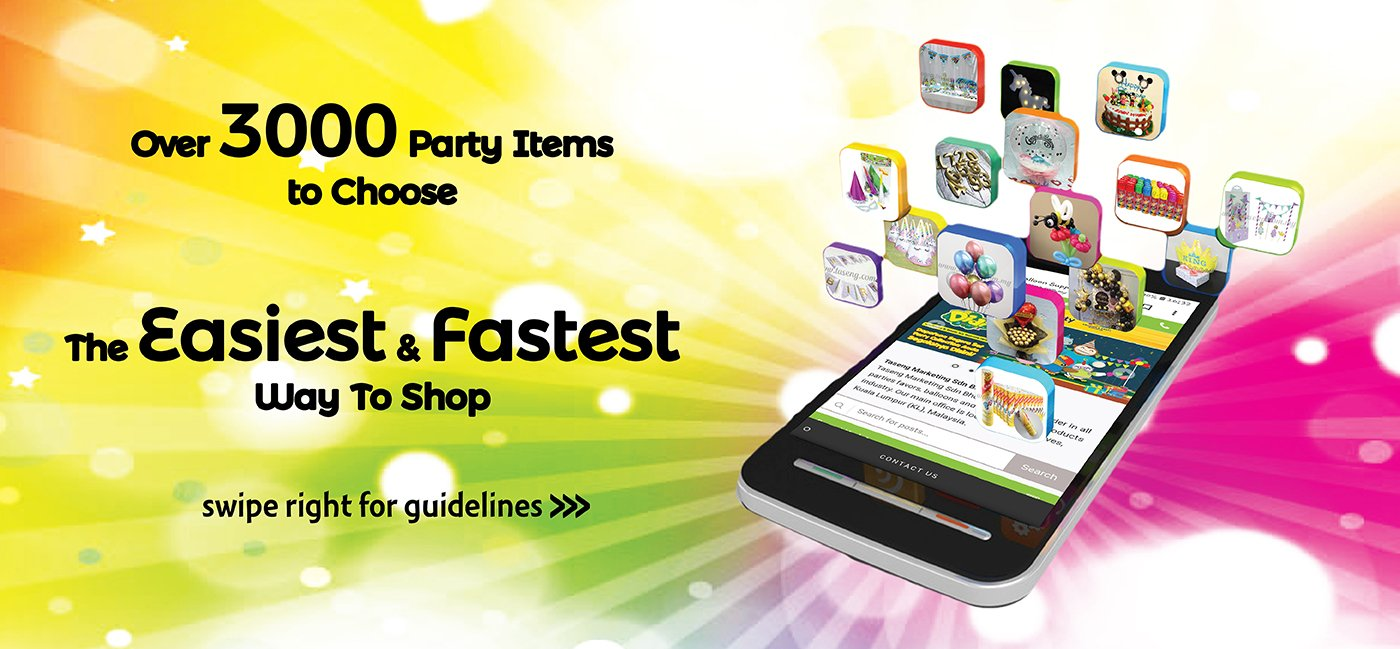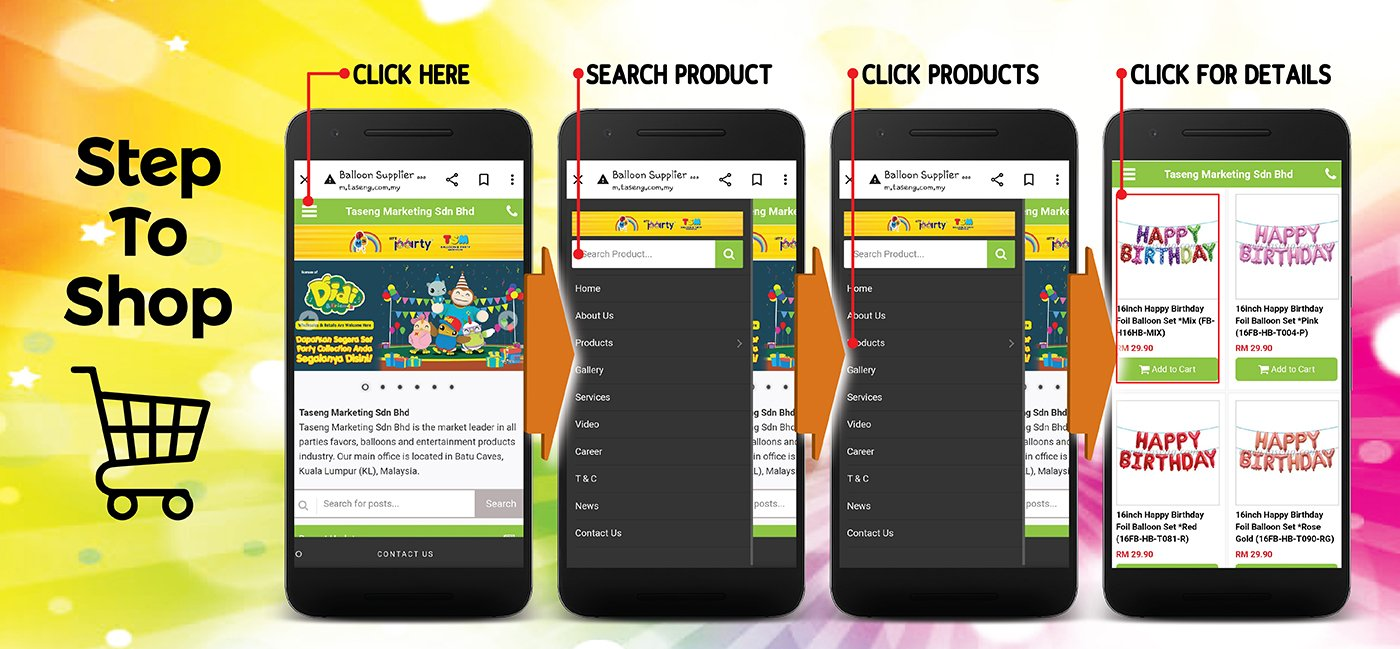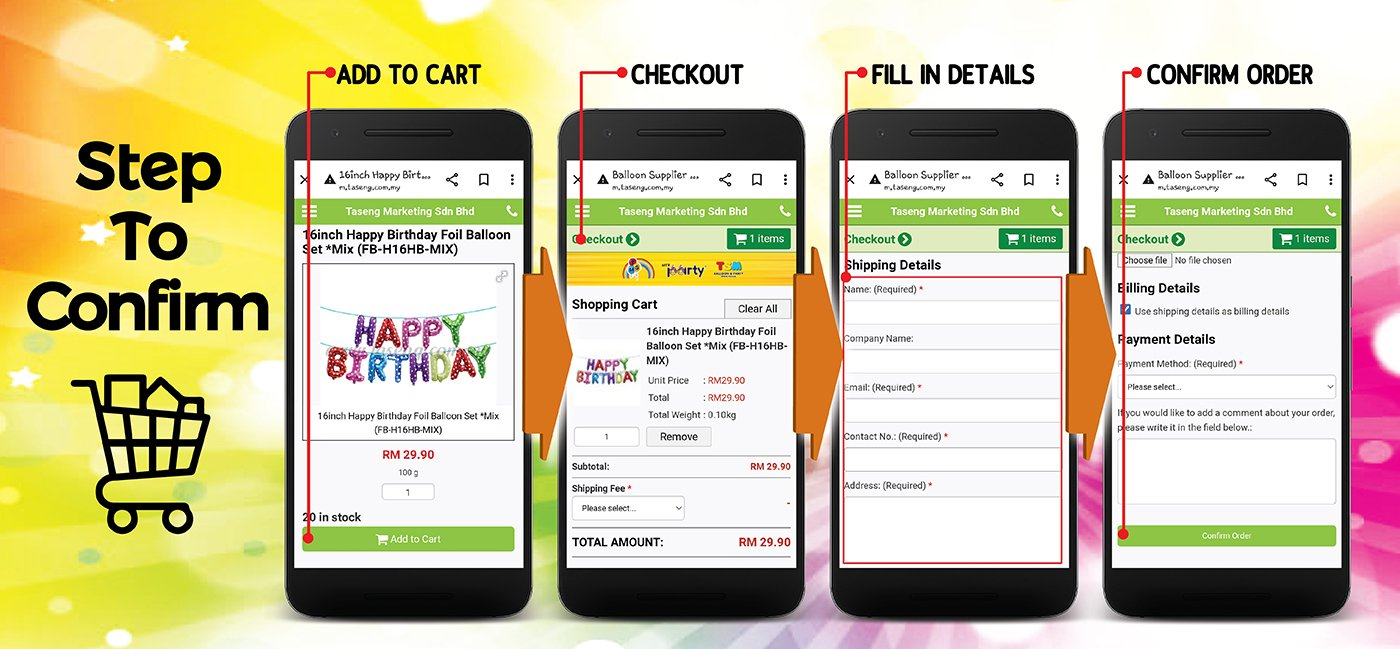## 36inch Standard Round Balloon### 36inch Metallic Round Balloon - Gold (B-36MR-M2)### 36inch Metallic Round Balloon - Gold 10pcs (B-36MR-M2)### 36inch Metallic Round Balloon - Silver (B-36MR-M1)### 36inch Metallic Round Balloon - Silver 10pcs (B-36MR-M1)### 36inch Standard Round Balloon - Black (B-36SR-ST8)### 36inch Standard Round Balloon - Black 10pcs (B-36SR-ST8)### 36inch Standard Round Balloon - Blue (B-36SR-ST2)### 36inch Standard Round Balloon - Blue 10pcs (B-36SR-ST2)### 36inch Standard Round Balloon - Clear (B-36SR-CR1)### 36inch Standard Round Balloon - Clear 10pcs (B-36SR-CR1)### 36inch Standard Round Balloon - Lavender (B-36SR-ST10)### 36inch Standard Round Balloon - Lavender 10pcs (B-36SR-ST10)### 36inch Standard Round Balloon - Lemon Green (B-36SR-ST6)### 36inch Standard Round Balloon - Lemon Green 10pcs (B-36SR-ST6)### 36inch Standard Round Balloon - Light Blue (B-36SR-ST9)### 36inch Standard Round Balloon - Light Blue 10pcs (B-36SR-ST9)### 36inch Standard Round Balloon - Orange (B-36SR-ST5)### 36inch Standard Round Balloon - Orange 10pcs (B-36SR-ST5)### 36inch Standard Round Balloon - Pink (B-36SR-ST3)### 36inch Standard Round Balloon - Pink 10pcs (B-36SR-ST3)### 36inch Standard Round Balloon - Red (B-36SR-ST7)### 36inch Standard Round Balloon - Red 10pcs (B-36SR-ST7)### 36inch Standard Round Balloon - White (B-36SR-ST1)### 36inch Standard Round Balloon - White 10pcs (B-36SR-ST1)